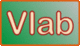online_amortization_1 calculator:   Monthly payments

 r' = (0.01 r)/12 A = P r'(1 + r')n/[(1 + r')n - 1] P = Initial loan amount (USD) r = Interest rate, annual (%) n = Total number of monthly payments A = Monthly payment (USD)INPUT:
Initial loan amount P (USD) :

Interest rate r, annual (%):

Total number of monthly payments n :

 OUTPUT:

Monthly payment A :   0.00

Request processed at 09:23:53 am on July 5th, 2022  [ 220705  09:23:53 ].

Thank you for running online_amortization_1